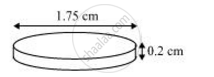# How many silver coins, 1.75 cm in diameter and of thickness 2 mm, must be melted to form a cuboid of dimensions 5.5 cm × 10 cm × 3.5 cm? - Mathematics

#### Question

How many silver coins, 1.75 cm in diameter and of thickness 2 mm, must be melted to form a cuboid of dimensions 5.5 cm × 10 cm × 3.5 cm? [Use  π=22/7]

#### SolutionCoins are cylindrical in shape.

Height (h1) of cylindrical coins = 2 mm = 0.2 cm

Radius (r) of circular end of coins = 1.75/2 =0.875 cm

Let n coins be melted to form the required cuboids.

Volume of n coins = Volume of cuboids

nxπxr2xh1 = lxbxh

n x π x (0.875)2 x 0.2 = 5.5 x 10 x 3.5

n = (5.5xx10xx3.5xx7)/((0.875)^2xx0.2xx22) = 400

Therefore, the number of coins melted to form such a cuboid is 400.

Is there an error in this question or solution?

#### APPEARS IN

NCERT Solution for Mathematics Textbook for Class 10 (2019 (Latest))
Chapter 13: Surface Areas and Volumes
13.3 | Q: 6 | Page no. 251

#### Video TutorialsVIEW ALL 

How many silver coins, 1.75 cm in diameter and of thickness 2 mm, must be melted to form a cuboid of dimensions 5.5 cm × 10 cm × 3.5 cm? Concept: Conversion of Solid from One Shape to Another.